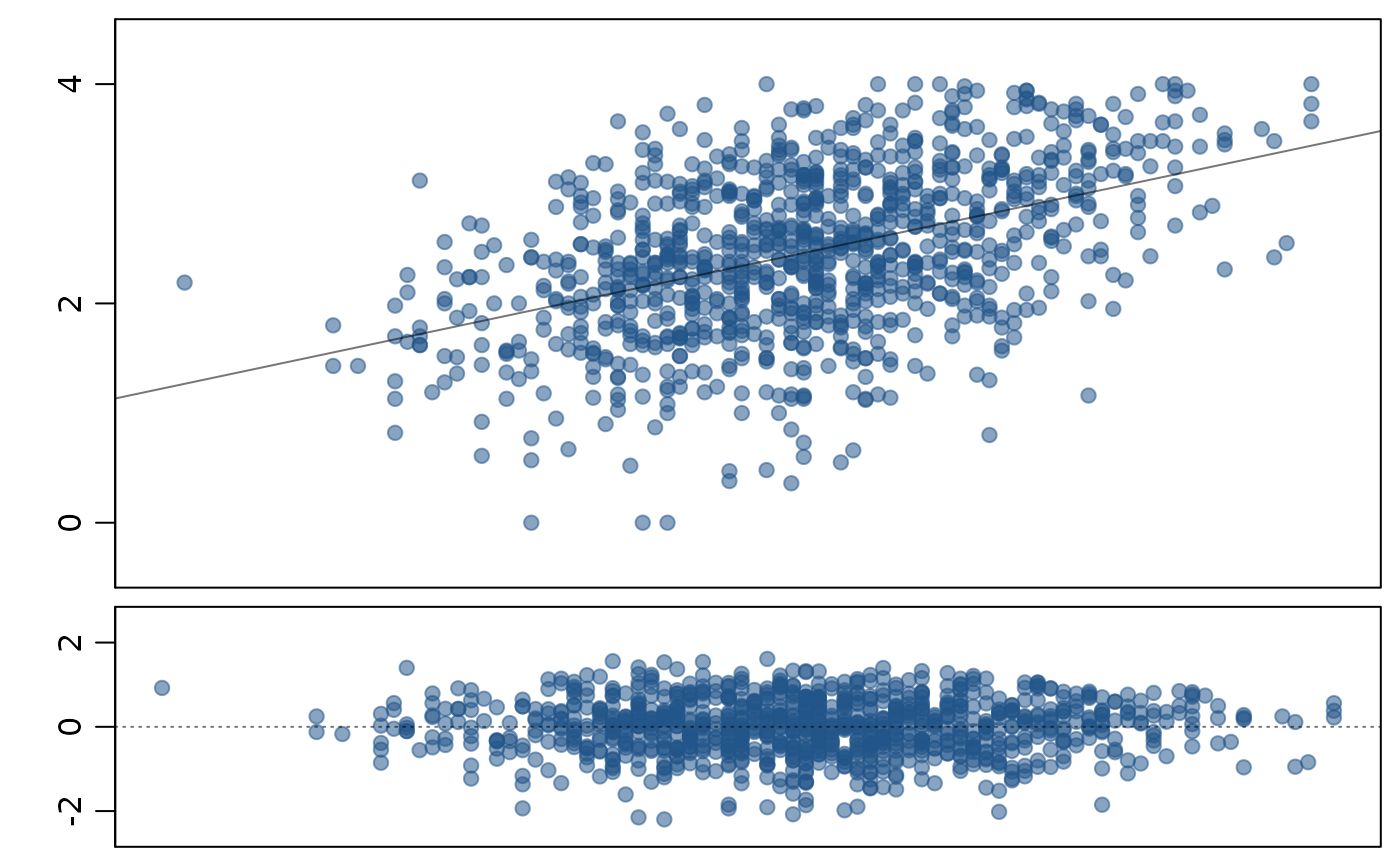Plot data, the linear model, and a residual plot simultaneously.

## Usage

lmPlot(
x,
y,
xAxis = 0,
yAxis = 4,
resAxis = 3,
resSymm = TRUE,
wBox = TRUE,
wLine = TRUE,
lCol = "#00000088",
lty = 1,
lwd = 1,
xlab = "",
ylab = "",
marRes = NULL,
col = "#22558888",
pch = 20,
cex = 1.5,
xR = 0.02,
yR = 0.1,
xlim = NULL,
ylim = NULL,
subset = NULL,
parCustom = FALSE,
myHeight = c(1, 0.45),
plots = c("both", "mainOnly", "resOnly"),
highlight = NULL,
hlCol = NULL,
hlCex = 1.5,
hlPch = 20,
na.rm = TRUE,
...
)

## Arguments

x

The x coordinates of points in the plot.

y

The y coordinates of points in the plot.

xAxis

The maximum number of x axis labels.

yAxis

The maximum number of y axis labels.

resAxis

The maximum number of y axis labels in the residual plot.

resSymm

Boolean determining whether the range of the residual plot should be symmetric about zero.

wBox

Boolean determining whether a box should be added around each plot.

wLine

Boolean determining whether to add a regression line to the plot.

lCol

The color of the regression line to be added.

lty

The line type of the regression line to be added.

lwd

The line width of the regression line to be added.

xlab

A label for the x axis.

ylab

A label for the y axis

marRes

Margin specified for the residuals.

col

Color of points.

pch

Plotting character.

cex

Plotting character size.

xR

Scaling the limits of the x axis. Ignored if xlim specified.

yR

Scaling the limits of the y axis. Ignored if ylim specified.

xlim

Limits for the x axis.

ylim

Limits for the y axis.

subset

A subset of the data to be used for the linear model.

parCustom

If TRUE, then the plotting margins are not modified automatically. This value should also be TRUE if the plots are being placed within a plot of multiple panels.

myHeight

A numerical vector of length 2 representing the ratio of the primary plot to the residual plot, in height.

plots

Not currently utilized.

highlight

Numerical vector specifying particular points to highlight.

hlCol

Color of highlighted points.

hlCex

Size of highlighted points.

hlPch

Plotting characters of highlighted points.

na.rm

Remove cases with NA values.

...

Additional arguments to plot.

makeTube

David Diez

## Examples


lmPlot(satgpa$sat_sum, satgpa$fy_gpa)lmPlot(gradestv$tv, gradestv$grades,
xAxis = 4,
xlab = "time watching TV", yR = 0.2, highlight = c(1, 15, 20)
)